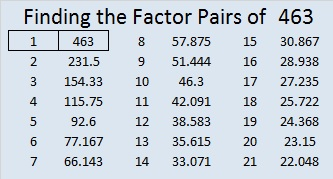# 463 and Level 5

463 is the sum of consecutive primes, too! Check the comments to see if any of my readers finds out what those consecutive primes are.

This Level 5 puzzle might be a little harder than usual. If you’ve solved a Level 5 puzzle before, see if you can meet this challenge!Print the puzzles or type the solution on this excel file:  10 Factors 2015-04-13

—————————————————————————————————

• 463 is a prime number.
• Prime factorization: 463 is prime.
• The exponent of prime number 463 is 1. Adding 1 to that exponent we get (1 + 1) = 2. Therefore 463 has exactly 2 factors.
• Factors of 463: 1, 463
• Factor pairs: 463 = 1 x 463
• 463 has no square factors that allow its square root to be simplified. √463 ≈ 21.5174How do we know that 463 is a prime number? If 463 were not a prime number, then it would be divisible by at least one prime number less than or equal to √463 ≈ 21.5174. Since 463 cannot be divided evenly by 2, 3, 5, 7, 11, 13, 17, or 19, we know that 463 is a prime number.

—————————————————————————————————## 6 thoughts on “463 and Level 5”

1.abyssbrain

They are actually pretty fun to find 🙂

53 + 59 + 61 + 67 + 71 + 73 + 79 = 463

•ivasallay

Yes, this is the type of fun most people never experience.

So far, at least person has met the challenge for the numbers 439, 440, 449, 457, 460, 462, and 463.

No one typed in the consecutive primes for 431 and 432 because I gave clues that made finding the primes not much of a challenge, but the comments are still waiting for someone to type in the consecutive primes that total 442 and 456 (two different ways).

•wbhs62

442’s OK; it’s 41+43+47+53+59+61+67+71

But I don’t have any solution for 456, let alone two!

•ivasallay

I’m glad 442 is OK now.

456 is even so unless one of the numbers is 2, the number of primes in the sum has to be an even number.

I will tell you that in both cases there are fewer than ten consecutive primes needed to make 456.

•abyssbrain

I’ll see if I can answer them 🙂

•ivasallay

By the time I saw this comment, you had found them all, too!

This site uses Akismet to reduce spam. Learn how your comment data is processed.